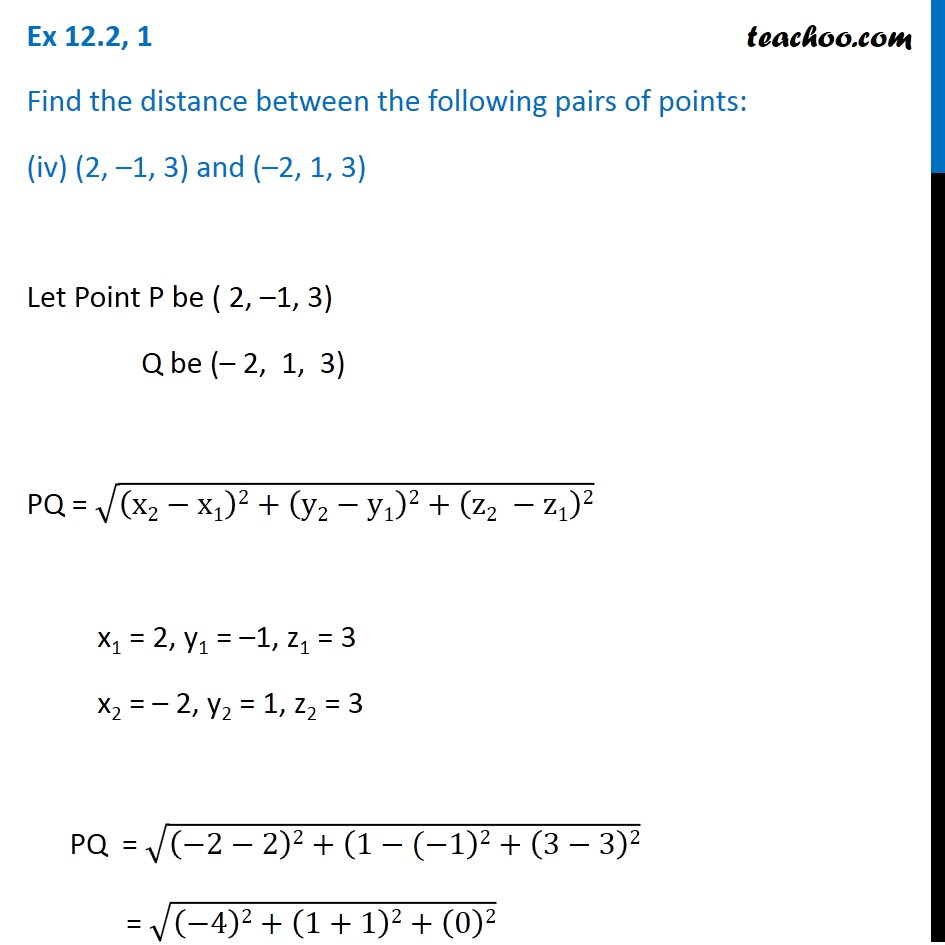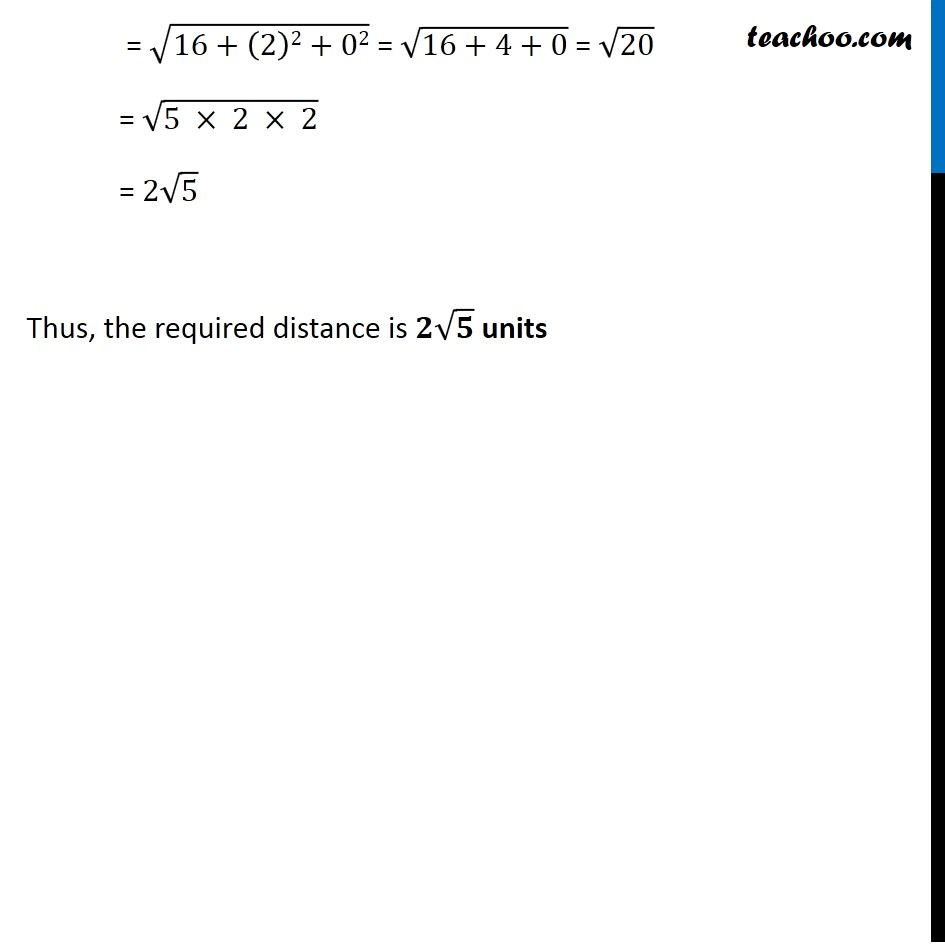Ex 11.2

Chapter 11 Class 11 - Intro to Three Dimensional Geometry
Serial order wiseLearn in your speed, with individual attention - Teachoo Maths 1-on-1 Class

### Transcript

Ex 11.2, 1 Find the distance between the following pairs of points: (iv) (2, –1, 3) and (–2, 1, 3) Let Point P be ( 2, –1, 3) Q be (– 2, 1, 3) PQ = √((x2−x1)2+(y2−y1)2+(z2 −z1)2) x1 = 2, y1 = –1, z1 = 3 x2 = – 2, y2 = 1, z2 = 3 PQ = √((−2−2)2+(1−(−1)2+(3−3)2) = √((−4)2+(1+1)2+(0)2) = √(16+(2)2+02) = √(16+4+0) = √20 = √(5×2×2) = 2√5 Thus, the required distance is 2√5 units = √(16+(2)2+02) = √(16+4+0) = √20 = √(5 × 2 × 2) = 2√5 Thus, the required distance is 𝟐√𝟓 units = √(16+(2)2+02) = √(16+4+0) = √20 = √(5 × 2 × 2) = 2√5 Thus, the required distance is 𝟐√𝟓 units = √(16+(2)2+02) = √(16+4+0) = √20 = √(5 × 2 × 2) = 2√5 Thus, the required distance is 𝟐√𝟓 units